# TI-30X Plus MathPrint™ scientific calculator

Advanced scientific calculator loaded with features that are intuitive to use. Probability calculations for the Normal, Binomial and Poisson distributions can be accessed through logical menus and prompts with no awkward syntax to memorise. List based entry for data and statistics makes it easier to enter and edit.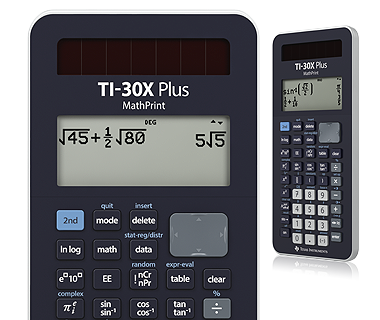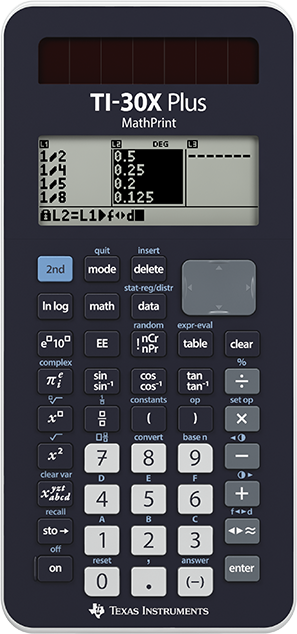## Key features

• Four line display
• One and Two variable statistics
• Nine different regression options
• Normal, Binomial and Poisson distributions
• Generate random samples, store results to a list and explore
• Unit conversions and scientific constants
• Prime Factorisation## View more calculations at a time

Four-line display allows you to enter more than one calculation, compare results and explore patterns, all on the same screen.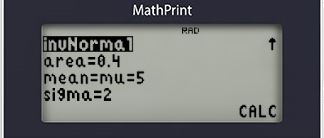## Probability distributions

Intuitive menus for Normal, inverse Normal, Binomial and Poisson distribution calculations, no messy syntax to memorise.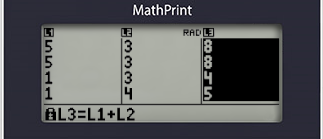## Store and explore

Generate random numbers for probability simulations and store them directly to a list. Explore the results as sums, products and more.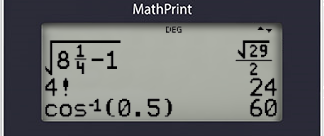## MathPrint™ feature

Use the MathPrint™ feature to display expressions, symbols, and fractions.## TI-30X Plus MathPrint™ scientific calculator specifications#### Built-in functionality

• Review and edit previous entries
• Random number and integer generator
• Normal, inverse Normal, Binomial and Poisson distributions
• Permutations and combinations
• One and two variable statistics
• List data entry and editing
• Constants – Speed of light, Gravity, Avogadro’s number and more
• Unit conversions – Temperature, speed, energy, pressure and more
• Expression definition, evaluation and tabulation
• Trigonometry and hyperbolic functions
• Complex numbers in polar and rectangular form
• Fractions, mixed, improper and decimal conversion
• Intuitive Menus and multi-tap keys
• EOS: (Equation Operating System)
• Scientific and engineering notation#### Hardware

• High resolution display with four lines of 16 characters
• Battery operation with the support of solar cells to extend battery life
• Automatic shutdown
• Hard plastic keys with colour coding
• Anti-slip rubber feet
• Robust cover with quick reference card#### Support## Extend the power of your calculator with accessories for the TI-30X Plus MathPrint™ scientific calculator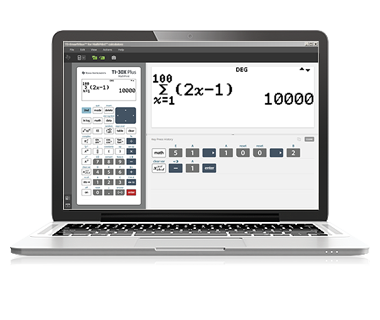## TI-SmartView™ emulator for MathPrint™ calculators

TI-SmartView™ emulator software for the TI-30X Plus MathPrint™ projects an interactive representation of the calculator and comes with unique instructional capabilities.

## Professional learning tutorials for teachers and students

Teachers can subscribe to our e-Newsletter to receive free resources and up to date information about workshops and webinars. Students (and teachers) can watch the free video tutorials on a range of topics and calculator functionality. Tutorials include free companion question sheets.

e-Newsletter student-getting-started

## Normal distribution – sample student tutorial

Calculate probabilities associated with the Normal distribution using the intuitive menu structure and prompts, no need to memorise awkward syntax.

## Binomial distribution – sample student tutorial

Calculate single probabilities or generate the entire distribution by storing the results in a list.

## Conditional probability – sample student tutorial

Make use of automatic storage and solve again options for simplicity and efficiency of calculations.